# Octal Number System

In early days octal number system was mostly used in minicomputers. The word “OCT” means eight. The octal number system says that it is a number system of base 8 which means that we require 8 different symbols in order to represent any number in octal system. The symbols are 0, 1, 2, 3, 4, 5, 6, and 7. The smallest two digit number in this system is (10)8 which is equivalent to decimal 8.
For example in this number system, the number is written as (352)8. The base should be written as 8 otherwise the number is assumed to be in decimal number system by default. So this thing needs to be taken care of in writing the number. A little error may result in the change in number system base. The main advantage of using octal number system is that it can be converted directly to binary in a very easy manner. As we know, the computer understands only the binary number system, so the conversion from binary to octal or from octal to binary is quite easier so this number system is used.

As its base is 8 = 23, every symbol of this system can be represented by its three bit binary equivalent.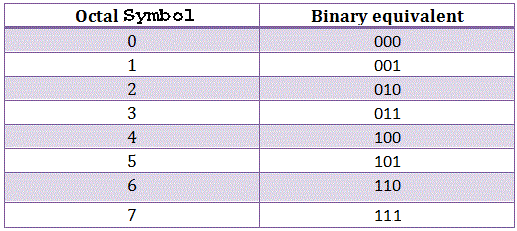As every digit of a number in octal system is represented separately by its three bit binary equivalent the octal system requires one-third of the length as compared to binary numbers. It is basically a positional weighted number system. The digit positions in octal number system has weight as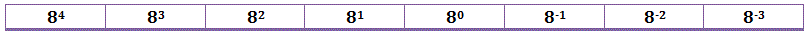## Number Conversion

### Octal to Binary Conversion

The conversion is done by converting an individual octal digit to binary. Every digit must be converted to a 3-bit binary number and the resultant will be the binary equivalent of an octal number.

Example
Converting (145.56)8 to binary-This table should be used in order to convert any octal number to binary. From the table, writing binary equivalent of each of the digit we get-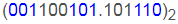which is the binary equivalent of the octal number.

### Binary to Octal Conversion

The same table can be used in order to convert a binary number to octal. First, group the binary number into the group of three bits and write the octal equivalent of it.

Example
Octal equivalent of (11001111)2 is
The groups we got here are-
011,001,111. A zero before the number is added in order to complete the grouping in the form of three binary digits.

Now the octal equivalent of the numbers are-
3, 1, 7. So the octal number we got is (317)8.

### Octal to Decimal Conversion

The method of converting an octal number into its decimal equivalent is very simple. Just expand the number in the base of eight with its positional weight and the resultant will be a decimal number.

Example
Converting (317)8 to its decimal equivalent.
This can be done as follows-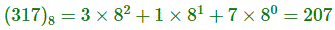### Decimal to Octal Conversion

This can be done by dividing the number by 8 using repeated division method known as double dabble method. The repeated division is done and the remainder is taken. It can be done as follows-

Example
Find the octal equivalent of 158.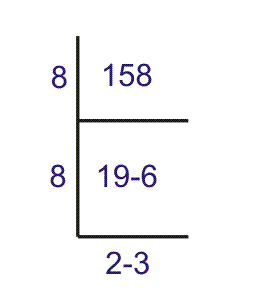The equivalent number in octal system is (236)8.
When there is a number in fraction or after the decimal point, that can be converted as-
Say we have to convert 0.40 to octal.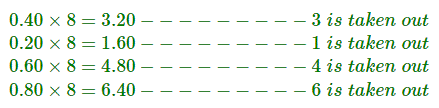So we see that the number is repeated. This will go on and it will be a never ending process so we can approximate the result as-
(.3146…)8.

## Advantages of Octal Number Systems

1. It is of one third length of the binary.
2. Easy conversion process from binary to octal and vice-versa.
3. Easier to handle input and output in the octal form.

## Disadvantages of Octal Number Systems

Computer does not understand octal number system so there must be a requirement of additional circuitry known as octal to binary converters before it is applied to a digital system or a computer.

Want To Learn Faster? 🎓
Get electrical articles delivered to your inbox every week.
No credit card required—it’s 100% free.